Custom SearchFAILURE ANALYSIS OF THE LC CAPACITOR-INPUT FILTER. - Shunt capacitors are subject to open circuits, short circuits, and excessive leakage; series inductors are subject to open windings and occasionally shorted turns or a short circuit to the core. The input capacitor (C1) has the greatest pulsating voltage applied to it, is the most susceptible to voltage surges, and has a generally higher average voltage applied. As a result, the input capacitor is frequently subject to voltage breakdown and shorting. The output capacitor (C2) is not as susceptible to voltage surges because of the series protection offered by the series inductor (L1), but the capacitor can become open, leaky, or shorted. A shorted capacitor, an open filter choke, or a choke winding which is shorted to the core, results in a no-output indication. A shorted capacitor, depending on the magnitude of the short, may cause a shorted rectifier, transformer, or filter choke, and may result in a blown fuse in the primary of the transformer. An open filter choke results in an abnormally high dc voltage at the input to the filter and no voltage at the output of the filter. A leaky or open capacitor in the filter circuit results in a low dc output voltage. This condition is generally accompanied by an excessive ripple amplitude. Shorted turns in the winding of a filter choke reduce the effective inductance of the choke and decrease its filtering efficiency. As a result, the ripple amplitude increases. VOLTAGE REGULATION Ideally, the output of most power supplies should be a constant voltage. Unfortunately, this is difficult to achieve. There are two factors that can cause the output voltage to change. First, the ac line voltage is not constant. The so-called 115 volts ac can vary from about 105 volts ac to 125 volts ac. This means that the peak ac voltage to which the rectifier responds can vary from about 148 volts to 177 volts. The ac line voltage alone can be responsible for nearly a 20 percent change in the dc output voltage. The second factor that can change the dc output voltage is a change in the load resistance. In complex electronic equipment, the load can change as circuits are switched in and out. In a television receiver, the load on a particular power supply may depend on the brightness of the screen, the control settings, or even the channel selected. These variations in load resistance tend to change the applied dc voltage because the power supply has a fixed internal resistance. If the load resistance decreases, the internal resistance of the power supply drops more voltage. This causes a decrease in the voltage across the load. Many circuits are designed to operate with a particular supply voltage. When the supply voltage changes, the operation of the circuit may be adversely affected. Consequently, some types of equipment must have power supplies that produce the same output voltage regardless of changes in the load resistance or changes in the ac line voltage. This constant output voltage may be achieved by adding a circuit called the VOLTAGE REGULATOR at the output of the filter. There are many different types of regulators in use today and to discuss all of them would be beyond the scope of this chapter. LOAD REGULATION A commonly used FIGURE OF MERIT for a power supply is its PERCENT OF REGULATION. The figure of merit gives us an indication of how much the output voltage changes over a range of load resistance values. The percent of regulation aids in the determination of the type of load regulation needed. Percent of regulation is determined by the equation: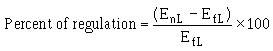This equation compares the change in output voltage at the two loading extremes to the voltage produced at full loading. For example, assume that a power supply produces 12 volts when the load current is zero. If the output voltage drops to 10 volts when full load current flows, then the percent of regulation is: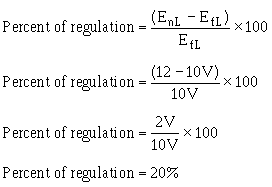Ideally, the output voltage should not change over the full range of operation. That is, a 12-volt power supply should produce 12 volts at no load, at full load, and at all points in between. In this case, the percent of regulation would be: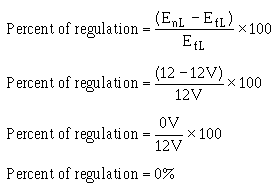Thus, zero-percent load regulation is the ideal situation. It means that the output voltage is constant under all load conditions. While you should strive for zero percent load regulation, in practical circuits you must settle for something less ideal. Even so, by using a voltage regulator, you can hold the percent of regulation to a very low value. REGULATORS You should know that the output of a power supply varies with changes in input voltage and circuit load current requirements. Because many electronic equipments require operating voltages and currents that must remain constant, some form of regulation is necessary. Circuits that maintain power supply voltages or current outputs within specified limits, or tolerances are called REGULATORS. They are designated as dc voltage or dc current regulators, depending on their specific application. Voltage regulator circuits are additions to basic power supply circuits, which are made up of rectified and filter sections (figure 4-30). The purpose of the voltage regulator is to provide an output voltage with little or no variation. Regulator circuits sense changes in output voltages and compensate for the changes. Regulators that maintain voltages within plus or minus (�) 0.1 percent are quite common. Figure 4-30. - Block diagram of a power supply and regulator.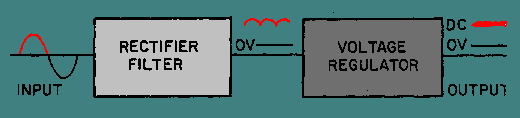Series and Shunt Voltage Regulators There are two basic types of voltage regulators. Basic voltage regulators are classified as either SERIES or SHUNT, depending on the location or position of the regulating element(s) in relation to the circuit load resistance. Figure 4-31 (view A and view B) illustrates these two basic types of voltage regulators. In actual practice the circuitry of regulating devices may be quite complex. Broken lines have been used in the figure to highlight the differences between the series and shunt regulators. Figure 4-31A. - Simple series and shunt regulators. SHUNT REGULATOR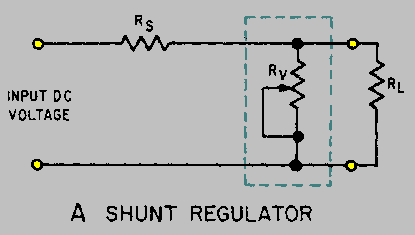Figure 4-31B. - Simple series and shunt regulators. SERIES REGULATOR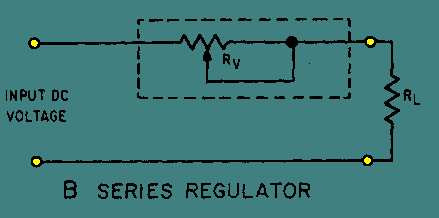The schematic drawing in view A is that of a shunt-type regulator. It is called a shunt-type regulator because the regulating device is connected in parallel with the load resistance. The schematic drawing in view B is that of a series regulator. It is called a series regulator because the regulating device is connected in series with the load resistance. Figure 4-32 illustrates the principle of series voltage regulation. As you study the figure, notice that the regulator is in series with the load resistance (RL) and that the fixed resistor (RS) is in series with the load resistance. Figure 4-32. - Series voltage regulator.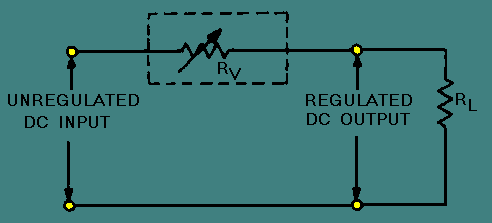You already know the voltage drop across a fixed resistor remains constant unless the current flowing through it varies (increases or decreases). In a shunt regulator, as shown in figure 4-33, output voltage regulation is determined by the current through the parallel resistance of the regulating device (RV), the load resistance (RL), and the series resistor (RS). For now, assume that the circuit is operating under normal conditions, that the input is 120 volts dc, and that the desired regulated output is 100 volts dc. For a 100-volt output to be maintained, 20 volts must be dropped across the series resistor (RS). If you assume that the value of RS is 2 ohms, you must have 10 amperes of current through RV and RL. (Remember: E = IR.) If the values of the resistance of RV and RL are equal, 5 amperes of current will flow through each resistance (RV and RL). Figure 4-33. - Shunt voltage regulator.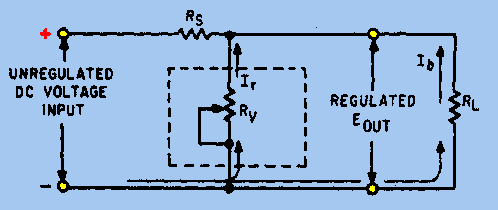Now, if the load resistance (RL) increases, the current through RL will decrease. For example, assume that the current through RL is now 4 amperes and that the total current through RS is 9 amperes. With this drop in current, the voltage drop across RS is 18 volts; consequently, the output of the regulator has increased to 102 volts. At this time, the regulating device (RV) decreases in resistance, and 6 amperes of current flows through this resistance (RV). Thus, the total current RS is once again 10 amperes (6 amperes through RV; 4 amperes through RL). Therefore, 20 volts is dropped across RS causing the output to decrease back to 100 volts. You should know by now that if the load resistance (RL) increases, the regulating device (RV) decreases its resistance to compensate for the change. If RL decreases, the opposite effect occurs and RV increases. Now consider the circuit when a decrease in load resistance takes place. When RL decreases, the current through RL subsequently increases to 6 amperes. This action causes a total of 11 amperes to flow through RS which then drops 22 volts. As a result, the output is 98 volts. However, the regulating device (RV) senses this change and increases its resistance so that less current (4 amperes) flows through RV. The total current again becomes 10 amperes, and the output is again 100 volts. From these examples, you should now understand that the shunt regulator maintains the desired output voltage first by sensing the current change in the parallel resistance of the circuit and then by compensating for the change. Again refer to the schematic shown in figure 4-33 and consider how the voltage regulator operates to compensate for changes in input voltages. You know, of course, that the input voltage may vary and that any variation must be compensated for by the regulating device. If an increase in input voltage occurs, the resistance of RV automatically decreases to maintain the correct voltage division between RV and RS. You should see, therefore, that the regulator operates in the opposite way to compensate for a decrease in input voltage. So far only voltage regulators that use variable resistors have been explained. However, this type of regulation has limitations. Obviously, the variable resistor cannot be adjusted rapidly enough to compensate for frequent fluctuations in voltages. Since input voltages fluctuate frequently and rapidly, the variable resistor is not a practical method for voltage regulation. A voltage regulator that operates continuously and automatically to regulate the output voltage without external manipulation is required for practical regulation. Q.28 Circuits which maintain constant voltage or current outputs are called dc voltage or dc current ___.Q.29 The purpose of a voltage regulator is to provide an output voltage with little or no ___.Q.30 The two basic types of voltage regulators are ___ and ___.Q.31 When a series voltage regulator is used to control output voltages, any increase in the input voltage results in an increase/a decrease (which one) in the resistance of the regulating device.Q.32 A shunt-type voltage regulator is connected in serial/parallel (which one) with the load resistance.